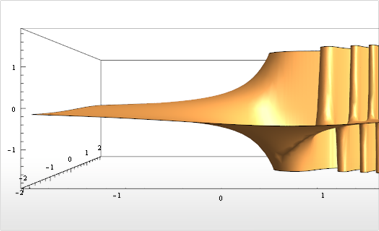# Enhanced Core Algorithms

Mathematica 9 adds a large number of improvements to existing functionality, as well as a smaller amount of new functionality. There are new special functions, such as real-valued algebraic functions from elementary calculus and Mittag-Leffler's E function from fractional calculus. Matrix functions are supplied for all of Mathematica's special functions and combinations. Numerous performance and scope improvements across the system have been added.• New real-valued cube root and surd functions.
• New Erlang B and C functions for capacity planning of queueing systems.
• New fractional calculus function: Mittag-Leffler's E function.
• Support for numeric, exact, and symbolic matrix functions.
• New graph-based algorithms for sparse exact and symbolic linear algebra.
• New high-performance algorithms for polynomial operations.
• New compact algebraic number representation for triangular polynomial systems.
• Ability to work large memory arrays and expressions only limited by 64-bit addressing.

## Mathematica

Questions? Comments? Contact a Wolfram expert »